Example Questions

← Previous 1

Example Question #1 : How To Multiply Fractions

What value of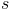makes the equation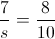true?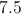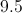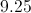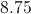Explanation:

We can simply cross multiply to obtain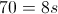and divide by 8 to solve for.

Example Question #2 : How To Multiply Fractions

Simplfiy the following expression;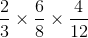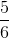Explanation:

Multiply the numerators  2 x 6 x 4 = 48. Then multiply the denominators 3 x 8 x 12 = 288.  The answer is 48/288. To simplify, divide both numerator and denominator by 48 to get 1/6.

Example Question #3 : How To Multiply Fractions

Evaluate –3–2 * 2–3.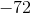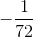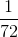Explanation:

Because the exponents are negative, we can convert –3–2  to 1/9 and 2–3 to 1/8. We then multiply straight across the top and the bottom, giving you 1/72.

Example Question #4 : How To Multiply Fractions

Simplify the following into one fraction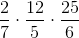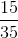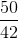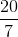Explanation:

To multiply fractions you multiply the entire numerator and the entire denominator together. However, before we do that we can cancel anything from the denominator with anything in the numerator.

Six cancels with 12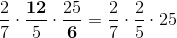5 cancels with 25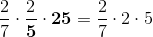multiply it all out and getExample Question #1 : How To Divide Fractions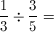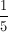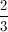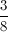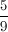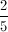Explanation:

Cross multiply or multiply using the reciprocal of the second fraction.

Example Question #1 : How To Divide Fractions

Simplify the following expression: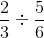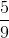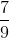Explanation: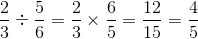Example Question #473 : Arithmetic

Evaluate the following: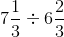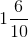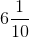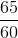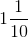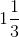Explanation:

Start by converting 71/to 22/3, and 6 2/to 20/3. We then multiply 22/3 by the reciprocal of 20/3, 3/20, and you get 66/60. This simplifies to 11/10.

Example Question #1 : How To Divide Fractions

Solve.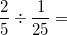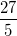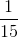Explanation:

Remember, to divide a number by a fraction, multiply the number by the reciprocal of the fraction. In this case,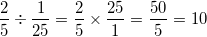Example Question #162 : Fractions

Solve for x where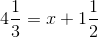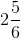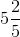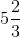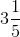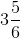Explanation:

To solve this, subtract 1 1/2 from both sides. Convert to common denominators.

4 1/3 – 1 1/2 = 4 2/6 – 1 3/6.

In order to subtract, you'll want to "borrow" from the 4 2/6. Rewrite 4 2/6 as 3 8/6 and then subtract 1 3/6 from this. Your solution is 2 5/6. Most calculators will also do these calculations for you.

Example Question #1 : How To Subtract Fractions

Solve for: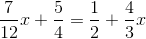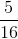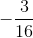Explanation: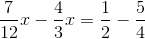Next, you need to find the common denominator. For the left side of your equation, it is. For the right, it is. This means that you need to rewrite as follows: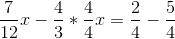Now, simplify and combine terms: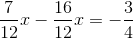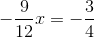You can further simplify the left side: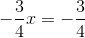Next, multiply both sides by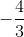. This gives you: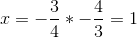← Previous 1